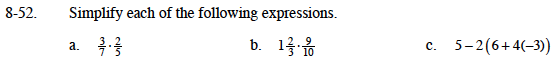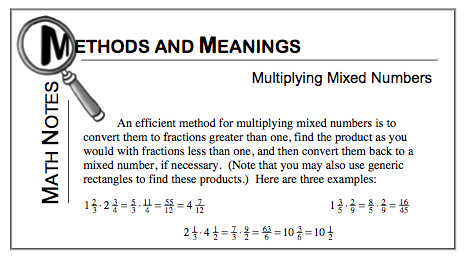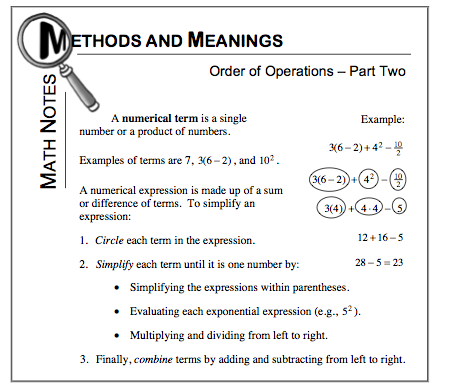### Home > MC1 > Chapter 8 > Lesson 8.2.3 > Problem8-52

8-52.For parts (a) and (b), refer to the Math Notes box below.Remember to follow the order of operations! Try circling the terms you recognize and then simplify.

Refer to the Math Notes box below for a reminder about the order of operations.$\frac{3}{2} \text{ or }1\frac{1}{2}.$

17

Were you able to find the answer to part (a)? Remember to show your work!